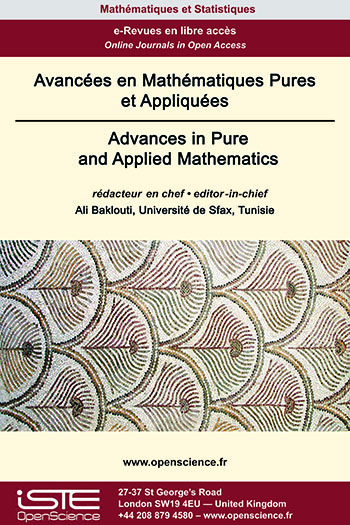# Vol 13 - Issue 2 (March 2022)

## List of Articles

Explicit formulas of the heat kernel on the quaternionic projective spaces

We consider the heat equation on the quaternionic projective space $P_{n}(ℍ)$, and we establish two formulas for the heat kernel, a series expansion involving Jacobi polynomials, and an integral representation involving a $\theta$-function. More precisely, using the quaternonic Hopf fibration and the explicit integral representation of the heat kernel on the complex projective space $P_{2n+1}(ℂ)$, as well as an integral representation of Jacobi polynomials in terms of Gegenbauer polynomials, we give an explicit integral representation of the heat kernel on the $n$-quaternionic projective space. We also establish an explicit series expansion of the heat kernel in terms of the Jacobi polynomials. Moreover, we derive an explicit formula of the heat kernel on the sphere $S^4$.

Weak Formulation of the Laplacian on the Full Shift Space

We consider a Laplacian on the one-sided full shift space over a finite symbol set, which is constructed as a renormalized limit of finite difference operators. We propose a weak de-nition of this Laplacian, analogous to the one in calculus, by choosing test functions as those which have finite energy and vanish on various boundary sets. In the abstract setting of the shift space, the boundary sets are chosen to be the sets on which the finite difference operators are defined. We then define the Neumann derivative of functions on these boundary sets and establish a relation between three important concepts in analysis so far, namely, the Laplacian, the bilinear energy form and the Neumann derivative of a function. As a result, we obtain the Gauss-Green’s formula analogous to the one in classical case. We conclude this paper by providing a sufficient condition for the Neumann boundary value problem on the shift space.

Weighted composition operators between two different weighted sequence spaces

We give simple criteria which characterize the symbols $u$, $\varphi$ defining continuous and compact weighted composition operators $W_{u,\varphi}$ acting between two different weighted sequence spaces. Also we characterize when $W_{u,\varphi}$ is bounded below and when it has closed range.

Attractors and chain recurrence for semigroup of continuous maps

In , we extended various notions of recurrence for the action of a semigroup analogous to their counterpart in the classical theory of dynamics. In this paper, we shall address the alternative de-nition of chain recurrent set in terms of attractors, given by Hurley in  following Conley’s characterization in . We shall also discuss the notion of topological transitivity and chain transitivity in this general setting.### Other issues :

2020

Volume 20- 11

Issue 1 (May 2020)
Issue 2 (September 2020)

2021

Volume 21- 12

Issue 1 (January 2021)
Issue 2 (May 2021)
Issue 3 (Special AUS-ICMS 2020)
Issue 4 (September 2021)

2022

Volume 22- 13

Forthcoming papers

Issue 1 (January 2022)
Issue 2 (March 2022)
Issue 3 (June 2022)
Issue 4 (September 2022)

2023

Volume 23- 14

Issue 1 (January 2023)
Issue 2 (Special CSMT 2022)